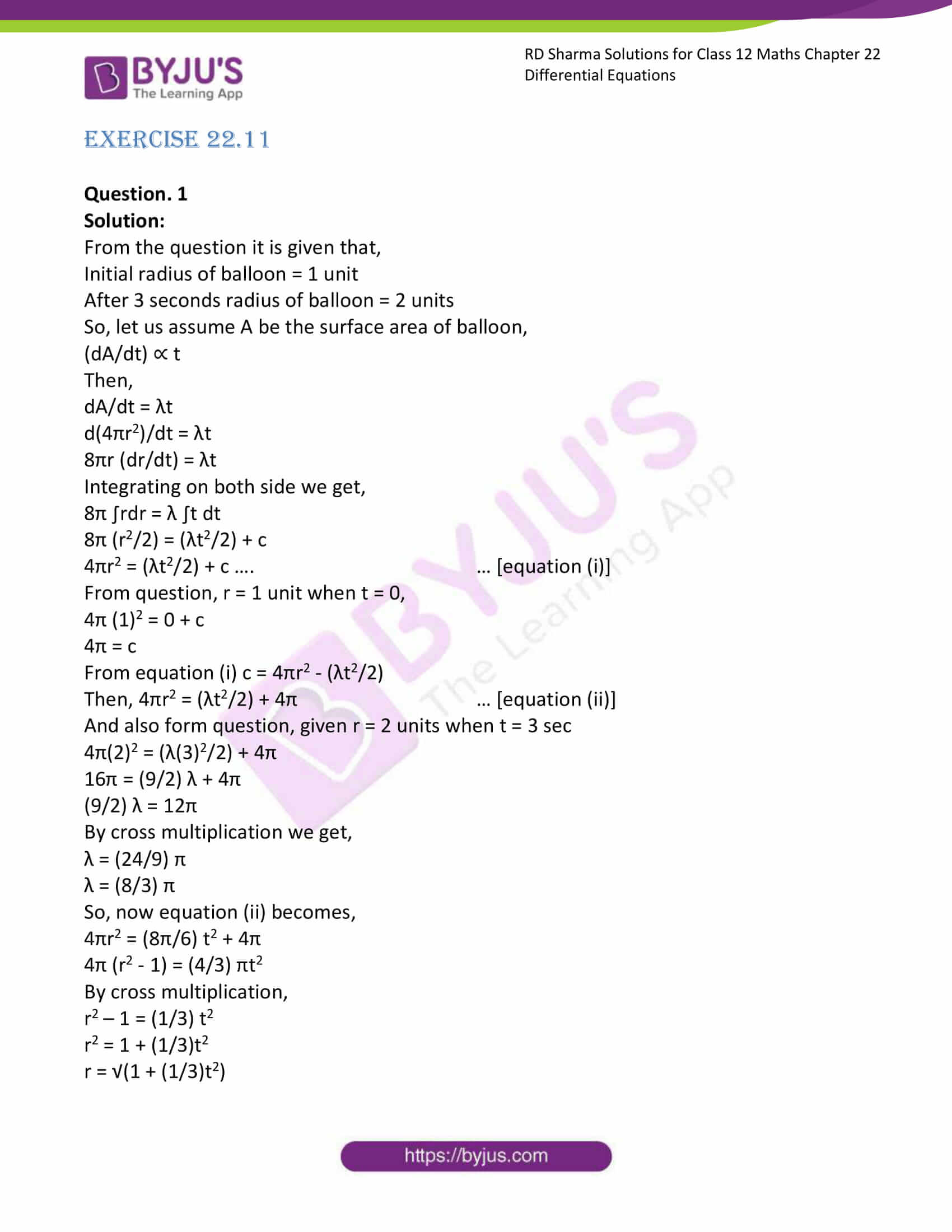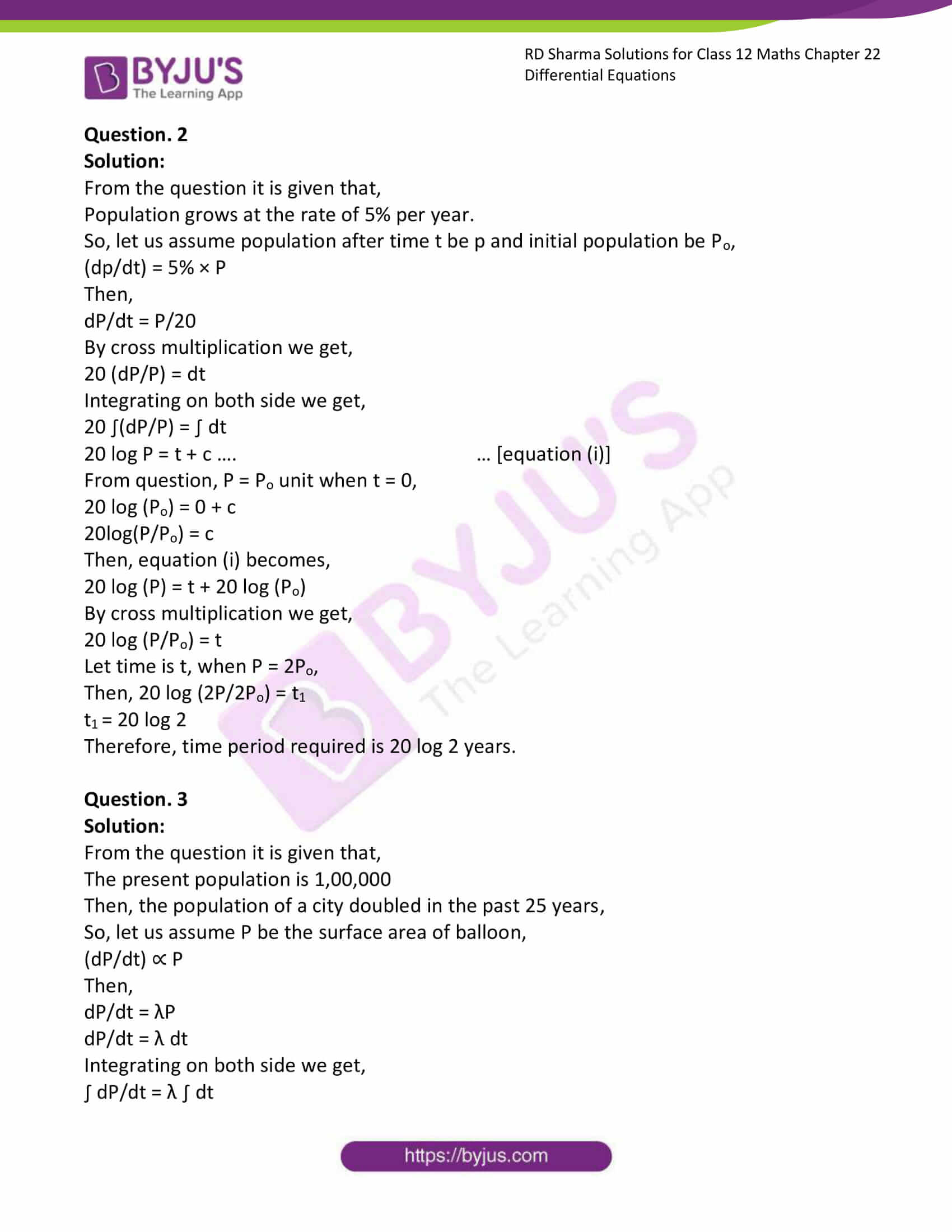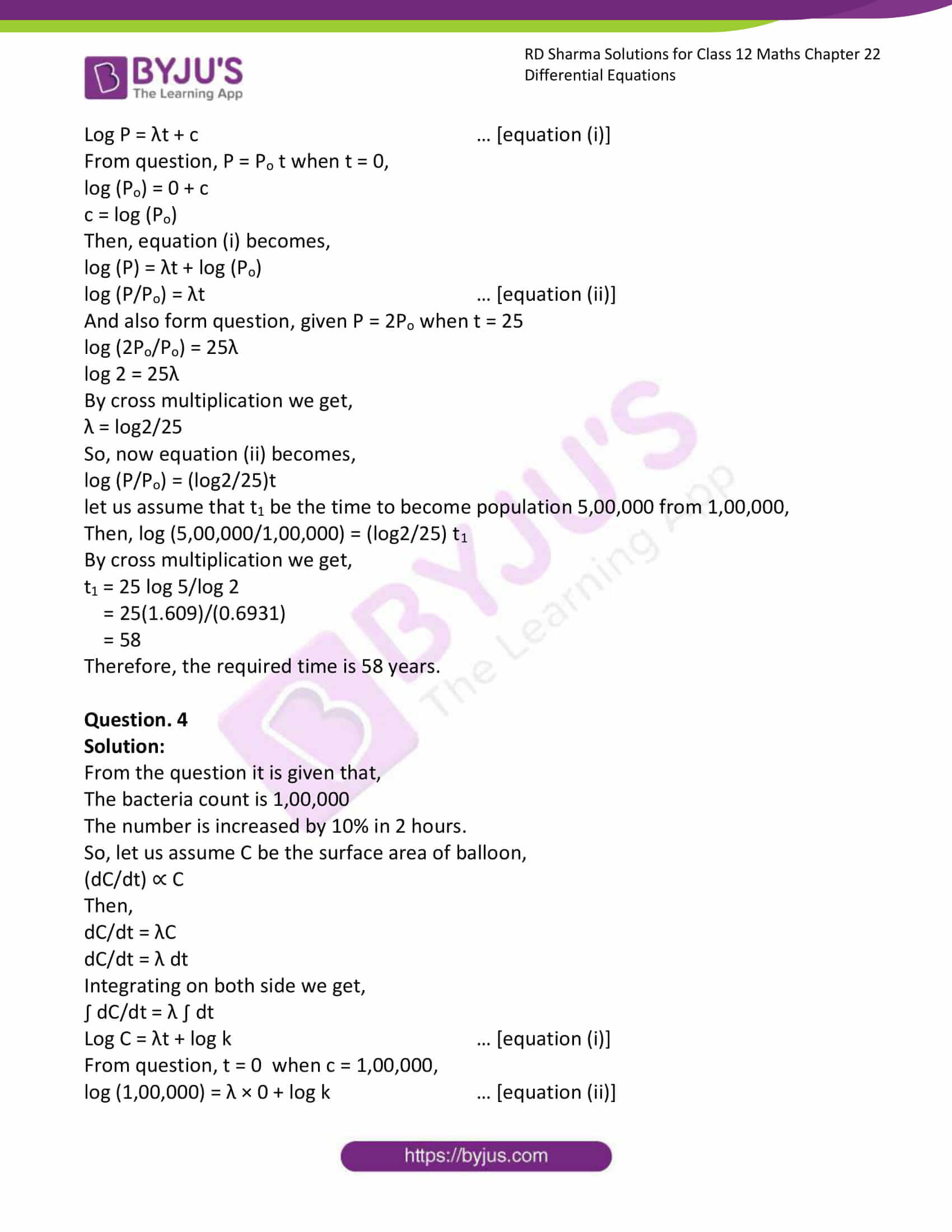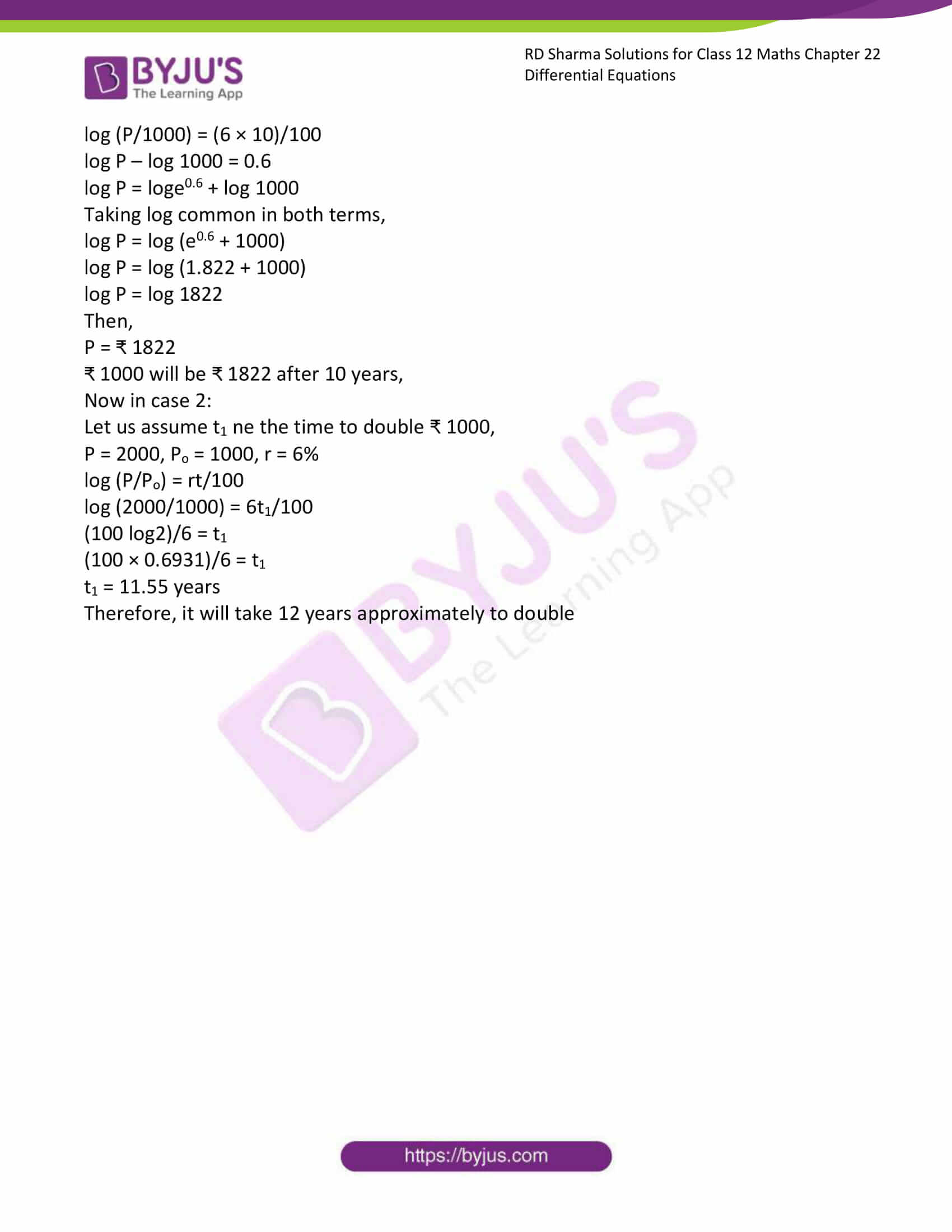# RD Sharma Solutions Class 12 Differential Equations Exercise 22.11

RD Sharma Solutions for Class 12 Maths Exercise 22.11 Chapter 22 Differential Equations are given here. Each question in these materials is related to the topics included in Class 12 CBSE syllabus and is designed by our experts to provide the best solutions to the problems, in which students face difficulties. Download RD Sharma Solutions for Class 12 Maths Chapter 22, in their respective links.

## Download PDF of RD Sharma Solutions for Class 12 Maths Chapter 22 Exercise 11### Access RD Sharma Solutions for Class 12 Maths Chapter 22 Exercise 11

EXERCISE 22.11

Question. 1

Solution:

From the question it is given that,

Initial radius of balloon = 1 unit

After 3 seconds radius of balloon = 2 units

So, let us assume A be the surface area of balloon,

(dA/dt) ∝ t

Then,

dA/dt = λt

d(4πr2)/dt = λt

8πr (dr/dt) = λt

Integrating on both side we get,

8π ∫rdr = λ ∫t dt

8π (r2/2) = (λt2/2) + c

4πr2 = (λt2/2) + c …. … [equation (i)]

From question, r = 1 unit when t = 0,

4π (1)2 = 0 + c

4π = c

From equation (i) c = 4πr2 – (λt2/2)

Then, 4πr2 = (λt2/2) + 4π … [equation (ii)]

And also form question, given r = 2 units when t = 3 sec

4π(2)2 = (λ(3)2/2) + 4π

16π = (9/2) λ + 4π

(9/2) λ = 12π

By cross multiplication we get,

λ = (24/9) π

λ = (8/3) π

So, now equation (ii) becomes,

4πr2 = (8π/6) t2 + 4π

4π (r2 – 1) = (4/3) πt2

By cross multiplication,

r2 – 1 = (1/3) t2

r2 = 1 + (1/3)t2

r = √(1 + (1/3)t2)

Question. 2

Solution:

From the question it is given that,

Population grows at the rate of 5% per year.

So, let us assume population after time t be p and initial population be Po,

(dp/dt) = 5% × P

Then,

dP/dt = P/20

By cross multiplication we get,

20 (dP/P) = dt

Integrating on both side we get,

20 ∫(dP/P) = ∫ dt

20 log P = t + c …. … [equation (i)]

From question, P = Po unit when t = 0,

20 log (Po) = 0 + c

20log(P/Po) = c

Then, equation (i) becomes,

20 log (P) = t + 20 log (Po)

By cross multiplication we get,

20 log (P/Po) = t

Let time is t, when P = 2Po,

Then, 20 log (2P/2Po) = t1

t1 = 20 log 2

Therefore, time period required is 20 log 2 years.

Question. 3

Solution:

From the question it is given that,

The present population is 1,00,000

Then, the population of a city doubled in the past 25 years,

So, let us assume P be the surface area of balloon,

(dP/dt) ∝ P

Then,

dP/dt = λP

dP/dt = λ dt

Integrating on both side we get,

∫ dP/dt = λ ∫ dt

Log P = λt + c … [equation (i)]

From question, P = Po t when t = 0,

log (Po) = 0 + c

c = log (Po)

Then, equation (i) becomes,

log (P) = λt + log (Po)

log (P/Po) = λt … [equation (ii)]

And also form question, given P = 2Po when t = 25

log (2Po/Po) = 25λ

log 2 = 25λ

By cross multiplication we get,

λ = log2/25

So, now equation (ii) becomes,

log (P/Po) = (log2/25)t

let us assume that t1 be the time to become population 5,00,000 from 1,00,000,

Then, log (5,00,000/1,00,000) = (log2/25) t1

By cross multiplication we get,

t1 = 25 log 5/log 2

= 25(1.609)/(0.6931)

= 58

Therefore, the required time is 58 years.

Question. 4

Solution:

From the question it is given that,

The bacteria count is 1,00,000

The number is increased by 10% in 2 hours.

So, let us assume C be the surface area of balloon,

(dC/dt) ∝ C

Then,

dC/dt = λC

dC/dt = λ dt

Integrating on both side we get,

∫ dC/dt = λ ∫ dt

Log C = λt + log k … [equation (i)]

From question, t = 0 when c = 1,00,000,

log (1,00,000) = λ × 0 + log k … [equation (ii)]

log (1,00,000) = log k … [equation (iii)]

And also form question, given t = 2, c = 1,00,000 + 1,00,000 × (10/100) = 110000

So, from equation (i) we have,

log 110000 = λ × 2 + log K … [equation (iv)]

Now, subtracting equation (ii) from equation (iv), we have,

Log 110000 – log 100000 = 2 λ

Then, log 11 × 10000 – log 10 × 10000 = 2 λ

Log ((11 × 10000)/(10 × 10000)) = 2λ

Log (11/10) = 2λ

So, λ = ½ log (11/10) … [equation (v)]

Now we need to find the time ‘t’ in which the count reaches 200000.

Then, substituting the values of λ and k from equation (iii) and (v) in equation (i),

Log 200000 = ½ log (11/10)t + log 100000

½ log (11/10)t = log 200000 – log 100000

½ log (11/10)t = log (200000/100000)

½ log(11/10)t = log 2

Therefore, the required time t = 2log2/log(11/10) hours.

Question. 5

Solution:

From the question it is given that,

The interest is compounded continuously at 6% per annum

So, let us assume P be the principal,

(dP/dt) = Pr/100

Then,

dP/dt = (r/100) dt

Integrating on both side we get,

∫ dP/P = ∫ r/100 dt

Log P = (rt/100) + c … [equation (i)]

Let us assume Po be the initial principal at t = 0

log (Po) = 0 + C

C = log (Po)

Now, substitute the value of C in equation (i)

log (P) = rt/100 + log (Po)

log (P/Po) = rt/100

Now in case 1:

Po = 1000, t = 10 years and r = 6

log (P/1000) = (6 × 10)/100

log P – log 1000 = 0.6

log P = loge0.6 + log 1000

Taking log common in both terms,

log P = log (e0.6 + 1000)

log P = log (1.822 + 1000)

log P = log 1822

Then,

P = ₹ 1822

₹ 1000 will be ₹ 1822 after 10 years,

Now in case 2:

Let us assume t1 ne the time to double ₹ 1000,

P = 2000, Po = 1000, r = 6%

log (P/Po) = rt/100

log (2000/1000) = 6t1/100

(100 log2)/6 = t1

(100 × 0.6931)/6 = t1

t1 = 11.55 years

Therefore, it will take 12 years approximately to double